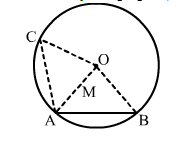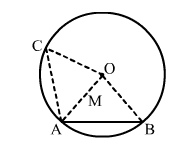# In the adjoining figure, AB and AC are two equal chords of a circle with centre O.

Question:

In the adjoining figure, AB and AC are two equal chords of a circle with centre O. Show that O lies on the bisector of ∠BAC.Solution:

Given: AB and AC are two equal chords of a circle with centre O.To prove: ∠OAB = ∠OAC
Construction: Join OA, OB and OC.
Proof:
In ΔOAB and ΔOAC, we have:
AB = AC         (Given)
OA = OA        (Common)
OB = OC         (Radii of a circle)
∴ Δ OAB  Δ OAC  (By SSS congruency rule)
⇒ ∠OAB = ∠OAC    (CPCT)
Hence, point lies on the bisector of ∠BAC.